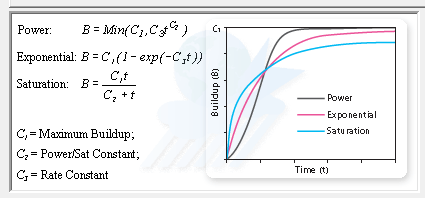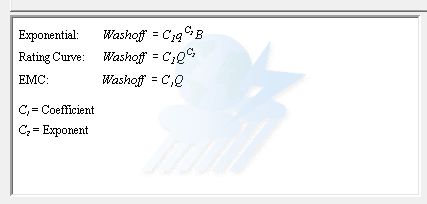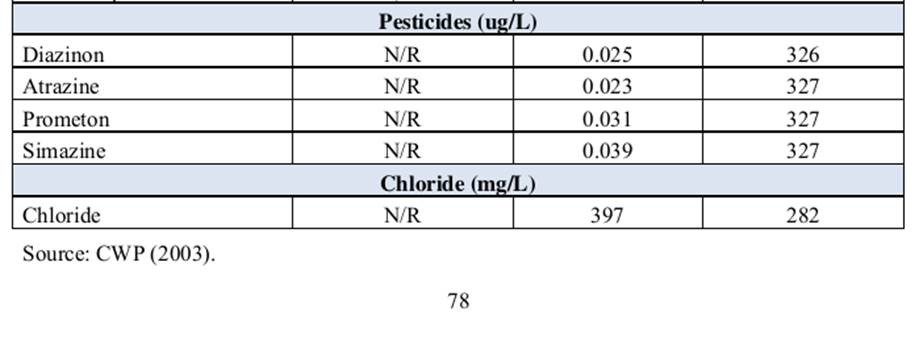SWMM5

# EPA SWMM 5 Water Quality

SWMM5 water quality can simulate the generation, inflow and transport of any number of user-defined pollutants. Required information for each pollutant includes:

 1 pollutant name
 2 concentration units (i.e., milligrams/liter, micrograms/liter, or counts/liter)
 3 concentration in rainfall
 4 concentration in groundwater
 5 concentration in inflow/infiltration
 6 concentration in dry weather flow
 7 initial concentration throughout the conveyance system
 8 first-order decay coefficient.

Co-pollutants can also be defined in SWMM5. For example, pollutant X can have a co-pollutant Y, meaning that the runoff concentration of X will have some fixed fraction of the runoff concentration of Y added to it.

Pollutant buildup and washoff from subcatchment areas are determined by the land uses assigned to those areas. Input loadings of pollutants to the drainage system can also originate from external time series inflows as well as from dry weather inflows.

The Pollutant Editor is invoked whenever a new Pollutant object is created or an existing pollutant is selected for editing. It contains the following fields:

Name

The name assigned to the pollutant.

Units

The concentration units (mg/L, ug/L, or #/L (counts/L)) in which the pollutant concentration is expressed.

Rain Concentration

Concentration of the pollutant in rain water (concentration units).

GW Concentration

Concentration of the pollutant in ground water (concentration units).

Initial Concentration

Concentration of the pollutant throughout the conveyance system at the start of the simulation.

I&I Concentration

Concentration of the pollutant in any Infiltration/Inflow (concentration units).

DWF Concentration

Concentration of the pollutant in any dry weather sanitary flow (concentration units). This value can be overridden for any specific node of the conveyance system by editing the node’s Inflows property.

Decay Coefficient

First-order decay coefficient of the pollutant (1/days).

Snow Only

YES if pollutant buildup occurs only when there is snow cover, NO otherwise (default is NO).

Co-Pollutant

Name of another pollutant whose runoff concentration contributes to the runoff concentration of the current pollutant.

Co-Fraction

Fraction of the co-pollutant’s runoff concentration that contributes to the runoff concentration of the current pollutant.

An example of a co-pollutant relationship would be where the runoff concentration of a particular heavy metal is some fixed fraction of the runoff concentration of suspended solids. In this case suspended solids would be declared as the co-pollutant for the heavy metal.

Land Uses are categories of development activities or land surface characteristics assigned to subcatchments. Examples of land use activities are residential, commercial, industrial, and undeveloped. Land surface characteristics might include rooftops, lawns, paved roads, undisturbed soils, etc. Land uses are used solely to account for spatial variation in pollutant buildup and washoff rates within subcatchments.

The SWMM user has many options for defining land uses and assigning them to subcatchment areas. One approach is to assign a mix of land uses for each subcatchment, which results in all land uses within the subcatchment having the same pervious and impervious characteristics. Another approach is to create subcatchments that have a single land use classification along with a distinct set of pervious and impervious characteristics that reflects the classification.

The following processes can be defined for each land use category:

Pollutant buildup that accumulates within a land use category is described (or “normalized”) by either a mass per unit of subcatchment area or per unit of curb length. Mass is expressed in pounds for US units and kilograms for metric units. The amount of buildup is a function of the number of preceding dry weather days and can be computed using one of the following functions:

Power Function

Pollutant buildup (B) accumulates proportional to time (t) raised to some power, until a maximum limit is achieved,where C1 = maximum buildup possible (mass per unit of area or curb length), C2 = buildup rate constant, and C3 = time exponent.

Exponential Function

Buildup follows an exponential growth curve that approaches a maximum limit asymptotically,where C1 = maximum buildup possible (mass per unit of area or curb length) and C2 = buildup rate constant (1/days).

Saturation Function

Buildup begins at a linear rate that continuously declines with time until a saturation value is reached,where C1 = maximum buildup possible (mass per unit area or curb length) and C2 = half-saturation constant (days to reach half of the maximum buildup).

External Time Series

This option allows one to use a Time Series to describe the rate of buildup per day as a function of time. The values placed in the time series would have units of mass per unit area (or curb length) per day. One can also provide a maximum possible buildup (mass per unit area or curb length) with this option and a scaling factor that multiplies the time series values.

Pollutant washoff from a given land use category occurs during wet weather periods and can be described in one of the following ways:

Exponential Washoff

The washoff load (W) in units of mass per hour is proportional to the product of runoff raised to some power and to the amount of buildup remaining, i.e.,where C1 = washoff coefficient, C2 = washoff exponent, q = runoff rate per unit area (inches/hour or mm/hour), and B = pollutant buildup in mass units. The buildup here is the total mass (not per area or per curb length) and both buildup and washoff mass units are the same as used to express the pollutant’s concentration (milligrams, micrograms, or counts).

Rating Curve Washoff

The rate of washoff W in mass per second is proportional to the runoff rate raised to some power, i.e.,where C1 = washoff coefficient, C2 = washoff exponent, and Q = runoff rate in user-defined flow units.

Event Mean Concentration

This is a special case of Rating Curve Washoff where the exponent is 1.0 and the coefficient C1 represents the washoff pollutant concentration in mass per liter. The conversion between user-defined flow units used for runoff and liters is handled internally by SWMM.Note that in each case buildup is continuously depleted as washoff proceeds, and washoff ceases when there is no more buildup available.

Washoff loads for a given pollutant and land use category can be reduced by a fixed percentage by specifying a BMP Removal Efficiency that reflects the effectiveness of any BMP controls associated with the land use. It is also possible to use the Event Mean Concentration option by itself, without having to model any pollutant buildup at all.Categories: SWMM5